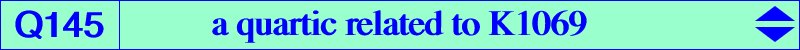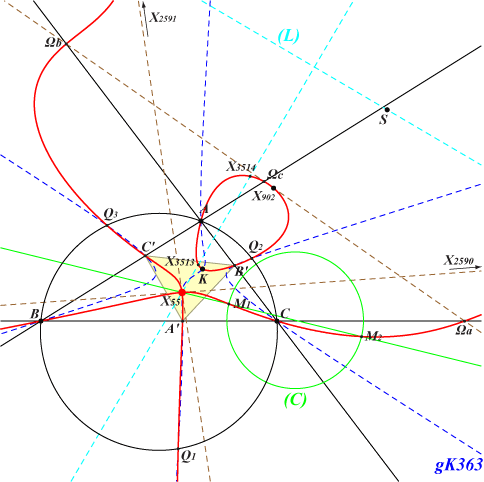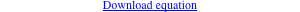too complicated to be written here. Click on the link to download a text file.X(6), X(55), X(902), X(2590), X(2591), X(3513), X(3514) vertices of the incentral triangle A'B'C' vertices of the tangential triangle KaKbKc centers Ωa, Ωb, Ωc of the Apollonius circles other points belowK1069 is the transform of the antiorthic axis i.e. the trilinear polar (L) of X(1) under the mapping 𝚹 : P -> aQ described in page K1065 where further informations and a generalization are given. The transform of Q145 is also K1069. Q145 is a nodal quartic analogous to Q098 and Q144. Its node is X(55) with nodal tangents passing through X(2590), X(2591) which also lie on the quartic. Note that the circle with diameter X(2590), X(2591) is orthogonal to the circumcircle (O) and passes through X(9), X(101), X(2291). Its center S is a^2 (b-c) (a^2-2 a b+b^2-2 a c+b c+c^2) : : , SEARCH = -8.86614652130293, on the antiorthic axis and on the lines {6,665}, {9,900}, {101,692}, etc. S is now X(22108) in ETC (2018-09-04). Q145 meets the circumcircle (O) at A, B, C, two imaginary points on the antiorthic axis and three real points Q1, Q2, Q3 on the isogonal transform of K363 = pK(X1, X2). Q145 meets the A-symmedian of ABC at A, K, Ka and a fourth point A1 which also lies on the line X(55)Ωa, B1 and C1 being defined likewise. A variable line passing through the node X(55) of Q145 meets Q145 again at two points M1, M2. The circle with diameter M1M2 is always orthogonal to the circumcircle (O) of ABC.